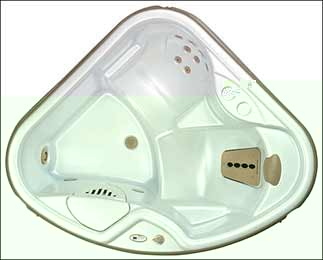Hot Spot TX:2006-2012  Hot Spot TX RX  or SX   is indicated by the letter in the serial number. for example.

Serial Number  TX RX SX-3H2015 the serial number would read TX RX SX is model  3 third quarter L year(2006) and 2015 is the unit number made.

Serial Number  TX RX SX-3J2015 the serial number would read TX RX SX is model  3 third quarter M year(2007) and 2015 is the unit number made.

Serial Number  TX RX SX-3K2015 the serial number would read TX RX SX is model  3 third quarter N year(2008) and 2015 is the unit number made.

Serial Number  TX RX SX-3L2015 the serial number would read TX RX SX is model  3 third quarter N year(2009) and 2015 is the unit number made

Serial Number  TX RX SX-3M2015 the serial number would read TX RX SX is model  3 third quarter N year(2010) and 2015 is the unit number made

Serial Number  TX RX SX -3N2015 the serial number would read TX RX SX  is model  3 third quarter N year(2011) and 2015 is the unit number made.

Serial Number  TX RX SX -3P2015 the serial number would read TX RX SX is model  3 third quarter N year(2012) and 2015 is the unit number made.

 Specifications Dimensions: 5’7" x 5’7" x 29" (1.7m x 1.7m x 0.74 m) Water Capacity: 130 gallons (492 liters) Weight: 316 lbs. (143 kg) dry 1,706 lbs. (774kg) filled Electrical Req: 115v, 15amp circuit 115v, 30amp circuit Ozonator: Not Available Filter: 71825 (Qty = 1)

HOT SPOT SX2006-/2012  Hot Spot TX  RX or SX   is indicated by the letter in the serial number. for example.

Serial Number  TX RX SX-3H2015 the serial number would read TX RX SX is model  3 third quarter L year(2006) and 2015 is the unit number made.

Serial Number  TX RX SX-3J2015 the serial number would read TX RX SX is model  3 third quarter M year(2007) and 2015 is the unit number made.

Serial Number  TX RX SX-3K2015 the serial number would read TX RX SX is model  3 third quarter N year(2008) and 2015 is the unit number made.

Serial Number  TX RX SX-3L2015 the serial number would read TX RX SX is model  3 third quarter N year(2009) and 2015 is the unit number made

Serial Number  TX RX SX-3M2015 the serial number would read TX RX SX is model  3 third quarter N year(2010) and 2015 is the unit number made

Serial Number  TX RX SX -3N2015 the serial number would TX or SX  is model  3 third quarter N year(2011) and 2015 is the unit number made.

Serial Number  TX  RX SX -3P2015 the serial number would read TX or SX is model  3 third quarter N year(2012) and 2015 is the unit number made.

 Specifications Dimensions: 6'6" x 6'6" x 33" (1.98m x 1.98m x .84m) Water Capacity: 285 gallons (1,079 liters) Weight: 439 lbs. (199 kg) dry 3,594 lbs. (1,630 kg) filled Electrical Req: 115v, 20amp circuit 115v, 30amp circuit Ozonator: FreshWater III Filter: 71825 (Qty = 1)

Below is a list of common replacement items. Be sure to see specifications page for parts diagrams or related Videos